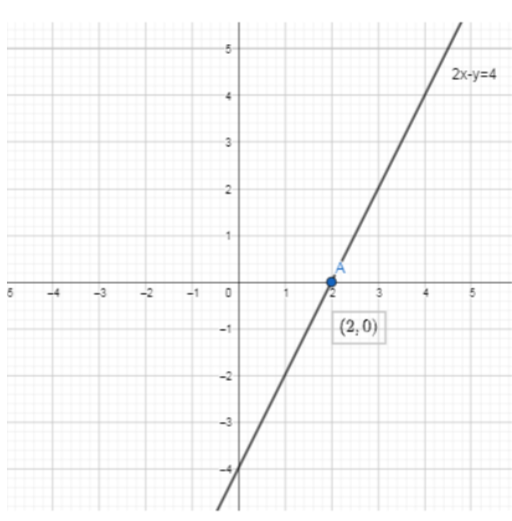QuestionAnswers

# Find the point where the graph of the linear equation $2x-y=4$ cuts the $x-\text{axis}$.(a) $\left( 2,0 \right)$(b) $\left( -2,0 \right)$(c) $\left( 0,-4 \right)$(d) $\left( 0,4 \right)$

Hint: In this question, in order to determine the point where the graph of the linear equation $2x-y=4$ cuts the$x-\text{axis}$, we have to find the $x-\text{intercept}$. For that we will have to find the value of $x$ by putting $y=0$ in the given linear equation $2x-y=4$. Suppose the value of $x$ is equal to say $a$ then the point $(a,0)$ is the required point at which the graph of the equation $2x-y=4$ cuts the x-axis.

Complete step-by-step solution:
The linear equation is given by $2x-y=4$.
In order to find the point where the graph of the give linear equation cuts the $x-\text{axis}$, we will first try to plot the graph of $2x-y=4$.
The graph of the linear equation is given byNow in order to find the point where the graph of the linear equation $2x-y=4$ cuts the x-axis, we have to find the $x-\text{intercept}$.
For that we have to find the value of $x$ by putting $y=0$ in the given linear equation $2x-y=4$.
Now by substituting the value $y=0$ in the linear equation $2x-y=4$, we get
$2x-y=4$
$\Rightarrow 2x-0=4$
$\Rightarrow 2x=4$
$\Rightarrow x=\dfrac{4}{2}$
$\Rightarrow x=2$
Thus, we get that the $x-\text{intercept}$ of the linear equation $2x-y=4$ is given by the point $\left( 2,0 \right)$.
Hence the graph of the linear equation $2x-y=4$ cuts the $x-\text{axis}$ at the point $\left( 2,0 \right)$.
Therefore option (a) is correct.

Note: In this problem, since we have to find the point at which linear equation $2x-y=4$ cuts the x-axis. thus the $y$ coordinate will always be zero. Do not make a mistake by taking the $x$ coordinate as zero otherwise the point will be on $y-\text{axis}$ and this will in term give the $y-\text{intercept}$ of the given linear equation.